﻿ 央行担保品框架下银行贷款的违约风险外移效应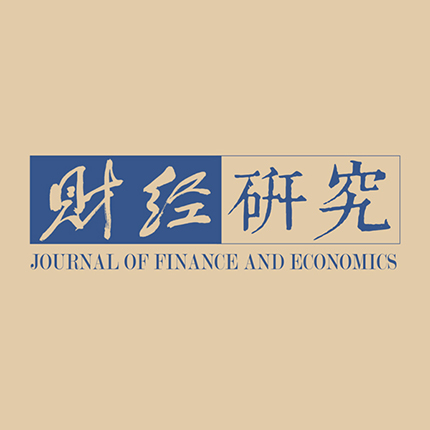《财经研究》
2023第49卷第5期

1. 上海财经大学 金融学院，上海 200433;
2. 上海财经大学 经济学院，上海 200433

Externalization Effect of Bank Loan Default Risk under the Central Bank Collateral Framework: Also on the Impact of Collateral Expansion on Macroeconomic Fluctuations and Monetary Policy Effectiveness
1. School of Finance, Shanghai University of Finance and Economics, Shanghai 200433, China;
2. School of Economics, Shanghai University of Finance and Economics, Shanghai 200433, China
Summary: In June 2018, the People’s Bank of China expanded its collateral framework by including credit assets of small and micro firms as eligible collateral. However, existing literature has explored the role of this policy in reducing corporate financing costs from the perspective of empirical analysis, while little studies its impact on macroeconomic fluctuations and monetary policy effectiveness. This paper extends the traditional financial accelerator model and constructs a new dynamic stochastic general equilibrium model that includes a central bank collateral framework. The conclusions of this paper are as follows: First, through the steady state analysis, it finds that central bank collateral expansion will encourage banks to externalize their loan default risk, thereby reducing corporate financing costs, and it will also increase the default risk of borrowing firms, reducing the steady state level of social welfare. Second, it analyzes the impact of central bank collateral expansion on macroeconomic fluctuations by comparing the impact of risk shocks and technology shocks before and after collateral expansion. The results show that, when the economy faces negative shocks, the externalization effect of default risk generated by collateral expansion will suppress the financial accelerator effect, thereby stabilizing the macro economy. Third, it discusses the impact of central bank collateral expansion on monetary policy effectiveness. The study finds that central bank collateral expansion can help to improve monetary policy effectiveness under quantity-based monetary policy rules, but it has little impact on the transmission efficiency of monetary policy under price-based monetary policy rules. Fourth, it extends the one-sector model to a two-sector model with state-owned firms and private firms, and uses the extended model to study the impact of including credit assets of small and micro firms as eligible collateral. The results show that, before collateral expansion, the expansion in lending facility is only beneficial to state-owned firms, and the cost of stimulating total output is the deterioration of resource allocation efficiency; after collateral expansion, the expansion in lending facility can stimulate both types of firm, but it has a greater stimulating effect on high-risk private firms. The main contributions of this paper are as follows: First, it introduces the central bank collateral framework into the financial accelerator model for the first time, which provides a model framework for analyzing the impact of this policy on the macro economy. Second, different from previous empirical analysis, it theoretically analyzes the externalization effect of bank loan default risk caused by including credit assets of small and micro firms as eligible collateral, and finds its role in stabilizing the macro economy. Third, it discusses the impact of central bank collateral expansion on monetary policy effectiveness, which has guiding significance for the practice of central bank monetary policy.
Key words: collateral framework    externalization effect of default risk    economic fluctuations    effectiveness of monetary policy

（一）最优债务合约

 $N+{B}_{t}^{N}={Q}_{t}{\bar{K}}^{N}_{t}$ (1)

 $\begin{array}{c}{\sigma }_{t}=\left(1-{\rho }_{\sigma }\right)\sigma +{\rho }_{\sigma }{\sigma }_{t-1}+{\varepsilon }_{\sigma t}\end{array}$ (2)

 $\begin{array}{c}{E}_{t}{{R}}_{t+1}^{k}={E}_{t}\dfrac{{r}_{t+1}^{k}{P}_{t+1}+\left(1-\delta \right){Q}_{t+1}}{{Q}_{t}}\end{array}$ (3)

 $\begin{array}{c}{\bar{\omega }}_{1,t+1}{{R}}_{t+1}^{k}\left(N+{B}_{t}^{N}\right)={Z}_{t+1}{B}_{t}^{N}\end{array}$ (4)

 $\begin{array}{c}{E}_{t}{\int }_{{\bar{\omega }}_{1,t+1}}^{\infty }{\omega }_{t+1}{{R}}_{t+1}^{k}\left(N+{B}_{t}^{N}\right)dF\left({\omega }_{t+1}\right)-{Z}_{t+1}{B}_{t}^{N}\left[1-F\left({\bar{\omega }}_{1,t+1}\right)\right]\end{array}$ (5)

 $\left({Z}_{t+1}{B}_{t}^{N}-{R}_{lt}{\tau }_{t}{B}_{t}^{N}\right)\left[1-F\left({\bar{\omega }}_{1,t+1}\right)\right] \begin{array}{c}+{\int }_{{\bar{\omega }}_{2,t+1}}^{{\bar{\omega }}_{1,t+1}}\left[\left(1-{\mu }_{1}\right){\omega }_{t+1}{{R}}_{t+1}^{k}\left({B}_{t}^{N}+N\right)-{R}_{lt}{\tau }_{t}{B}_{t}^{N}\right]dF\left({\omega }_{t+1}\right)\geqslant {R}_{t}\left(1-{\tau }_{t}\right){B}_{t}^{N}\end{array}$ (6)

 $\begin{array}{c}{\bar{\omega }}_{2,t+1}=\dfrac{{R}_{lt}{\tau }_{t}{B}_{t}^{N}}{\left(1-{\mu }_{1}\right){{R}}_{t+1}^{k}\left({B}_{t}^{N}+N\right)}\end{array}$ (7)

 $\begin{array}{c}{\int }_{0}^{{\bar{\omega }}_{2,t+1}}\left[{R}_{lt}{\tau }_{t}{B}_{t}^{N}-\left(1-{\mu }_{1}\right){\omega }_{t+1}{{R}}_{t+1}^{k}\left({B}_{t}^{N}+N\right)\right]dF\left({\omega }_{t+1}\right)\end{array}$ (8)

 $\begin{array}{c}{R}_{lt}{\tau }_{t}{B}_{t}^{N}\left[F\left({\bar{\omega }}_{2,t+1}\right)-{\int }_{0}^{{\bar{\omega }}_{2,t+1}}{\omega }_{t+1}/{\bar{\omega }}_{2,t+1}dF\left({\omega }_{t+1}\right)\right]\end{array}$ (9)

（二）中央银行与货币政策

 ${M}_{t}-{M}_{t-1}={B}_{gt}-{R}_{g,t-1}{B}_{g,t-1}+{M}_{lt}-{\int }_{{\bar{\omega }}_{2t}}^{\mathrm{\infty }}{R}_{l,t-1}{M}_{l,t-1}dF\left({\omega }_{t}\right) -{\int }_{0}^{{\bar{\omega }}_{2t}}\left(1-{\mu }_{1}\right){\omega }_{t}{{R}}_{t}^{k}\left({N}_{t-1}+{B}_{t-1}\right)dF\left({\omega }_{t}\right)$ (10)

 $\begin{array}{c}\ln\left(\dfrac{{g}_{mt}}{{g}_{m}}\right)={\rho }_{m}\ln\left(\dfrac{{g}_{m,t-1}}{{g}_{m}}\right)+{\psi }_{mp}\ln\left(\dfrac{{\pi }_{t}}{\pi }\right)+{\psi }_{my}\ln\left(\dfrac{{g}_{yt}}{{g}_{y}}\right)+{\varepsilon }_{mt}\end{array}$ (11)

（三）市场出清

 ${Y}_{t}^{f}= {C}_{t}+{I}_{t}+\dfrac{{\Omega }_{p}}{2}{\left(\dfrac{{\pi }_{t}}{\pi }-1\right)}^{2}{C}_{t}+{{R}}_{t}^{k}\dfrac{{N}_{t}+{B}_{t}}{{P}_{t}}{\mu }_{1}{\int }_{{\omega }_{2t}}^{{\bar{\omega }}_{1t}}{\omega }_{t}dF\left({\omega }_{t}\right) +{{R}}_{t}^{k}\dfrac{{N}_{t}+{B}_{t}}{{P}_{t}}{\mu }_{2}{\int }_{0}^{{\bar{\omega }}_{2t}}{\omega }_{t}dF\left({\omega }_{t}\right)$ (12)

 $\begin{array}{c}GD{P}_{t}={C}_{t}+{I}_{t}\end{array}$ (13)

 $\begin{array}{c}{B}_{t}={\tau }_{t}{B}_{t}+{D}_{t}\end{array}$ (14)

 $\begin{array}{c}{M}_{t}={D}_{t}+{M}_{t}^{H}\end{array}$ (15)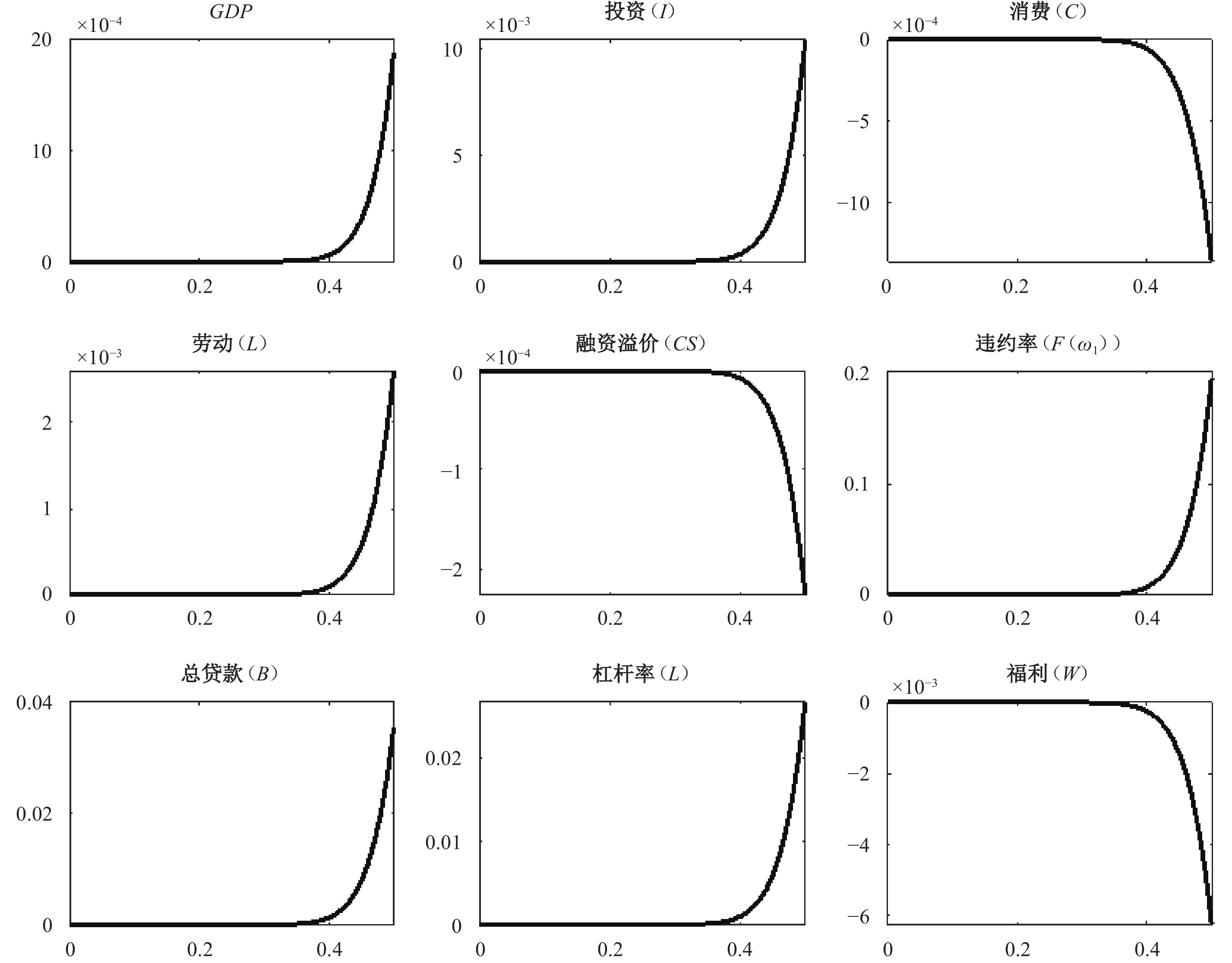图 1 信贷资产抵押率与关键变量稳态关系 注：纵轴表示各变量相对于零抵押率（$\tau =0$）下稳态的偏离百分比，横轴表示信贷资产抵押率。融资溢价${CS}_{t}={{E}_{t}{R}}_{t+1}^{k}/{R}_{t}$，杠杆率${L}_{t}=({B}_{t}+{N}_{t})/{N}_{t}$。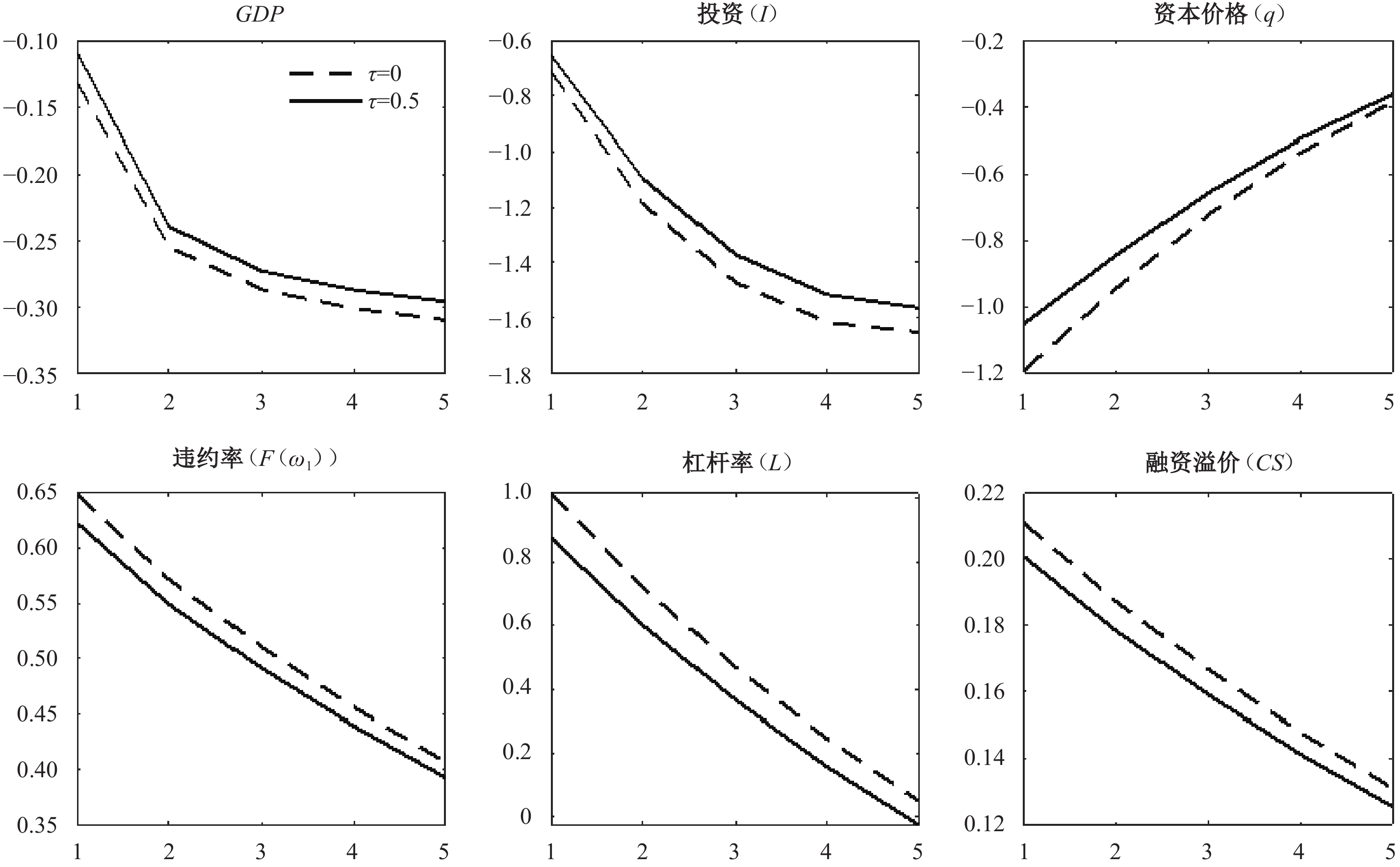图 2 不同稳态抵押率下风险冲击对经济的影响 注：风险冲击=1%。纵轴表示变量相对于稳态值的偏离百分比，横轴表示时间。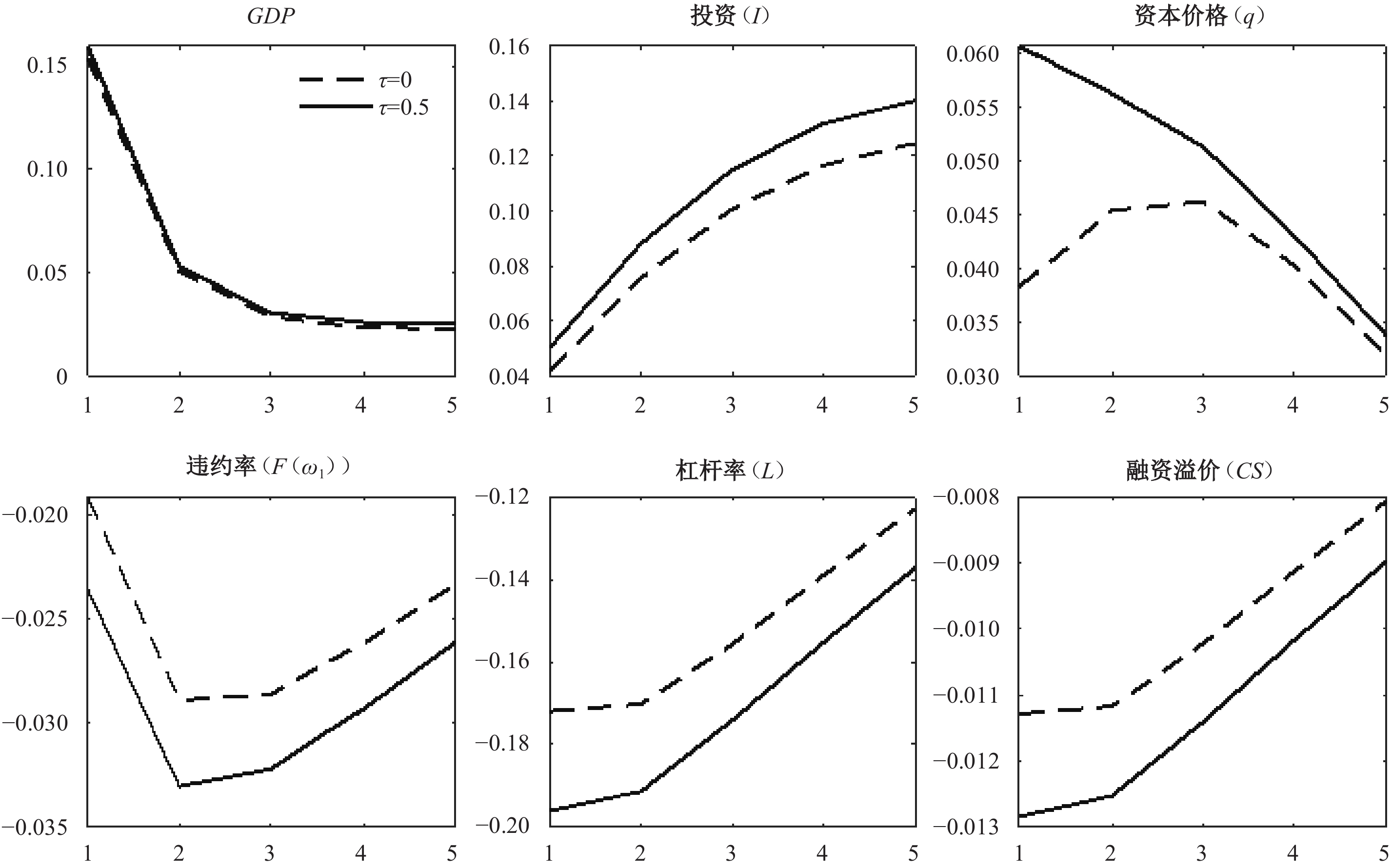图 3 不同稳态抵押率下货币供给正向冲击对经济的影响 注：货币供给冲击=1%。纵轴表示变量相对于稳态值的偏离百分比，横轴表示时间。

 $\begin{array}{c}\ln\left(\dfrac{{R}_{t}}{R}\right)={\rho }_{r}\ln\left(\dfrac{{R}_{t-1}}{R}\right)+{\psi }_{rp}\ln\left(\dfrac{{\pi }_{t}}{\pi }\right)+{\psi }_{ry}\ln\left(\dfrac{{g}_{yt}}{{g}_{y}}\right)+{\varepsilon }_{rt}\end{array}$ (16)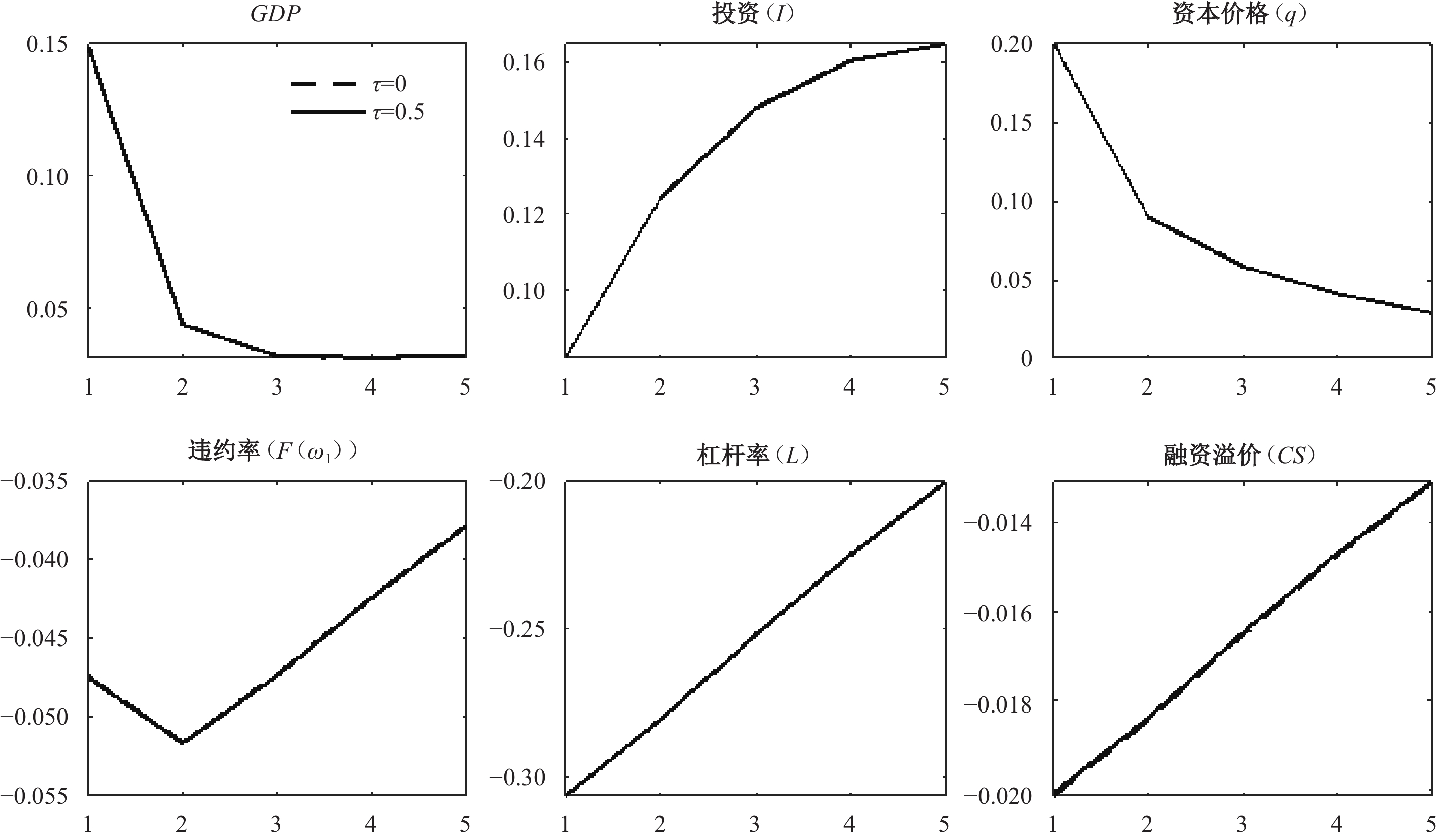图 4 不同稳态抵押率下降息冲击对经济的影响（Taylor规则） 注：利率冲击=−1%。纵轴表示变量相对于稳态值的偏离百分比，横轴表示时间。

（一）多企业部门模型

 $\begin{array}{c} \underset{{\bar{B}}_{it},{\bar{\omega }}_{1,t+1}^{i}}{\mathrm{m}\mathrm{a}\mathrm{x}} \;\;\; \text{}{E}_{t}{{R}}_{i,t+1}^{k}\left({\bar{N}}_{it}+{\bar{B}}_{it}\right)g\left({\bar{\omega }}_{1,t+1}^{i}\right) \\ \begin{array}{c} {\rm{s.t.}} \;\;\;{{R}}_{i,t+1}^{k}\left({\bar{N}}_{it}+{\bar{B}}_{it}\right)h\left({\bar{\omega }}_{1,t+1}^{i},{\bar{\omega }}_{2,t+1}^{i}\right)\ge {R}_{t}\left(1-{\tau }_{i}\right){\bar{B}}_{it}+{R}_{lt}{\tau }_{i}{\bar{B}}_{it}\left[1-F\left({\bar{\omega }}_{2,t+1}^{i}\right)\right]\end{array} \end{array}$ (17)
 $\begin{array}{c}{\bar{\omega }}_{2,t+1}^{i}=\dfrac{{R}_{lt}{\tau }_{i}{\bar{B}}_{it}}{\left(1-{\mu }_{i1}\right){{R}}_{i,t+1}^{k}\left({\bar{N}}_{it}+{\bar{B}}_{it}\right)}\end{array}$ (18)

（二）宏观经济波动分析

（三）借贷便利工具有效性分析

 $\begin{array}{c}{M}_{lt}={\tau }_{p}{B}_{pt}+{\tau }_{s}{B}_{st}\end{array}$ (19)

 $\begin{array}{c}{g}_{lt}=\left(1-{\rho }_{g}\right){g}_{l}+{\rho }_{g}{g}_{l,t-1}+{\varepsilon }_{gt}\end{array}$ (20)

 $\begin{array}{c}{R}_{lt}=\left(1-{\rho }_{l}\right){R}_{l}+{\rho }_{l}{R}_{l,t-1}+{\varepsilon }_{lt}\end{array}$ (21)图 5 不同私企信贷资产抵押率下借贷便利操作规模上升1%对经济的影响 注：纵轴表示变量相对于稳态值的偏离百分比，横轴表示时间。私企融资溢价${CS}_{pt}={{E}_{t}{R}}_{p,t+1}^{k}/{R}_{t}$，杠杆率${L}_{pt}=({B}_{pt}+{N}_{pt})/{N}_{pt}$。图 6 不同私企信贷资产抵押率下借贷便利操作利率下降1%对经济的影响 注：纵轴表示变量相对于稳态值的偏离百分比，横轴表示时间。

2018年6月中国人民银行决定将小微企业信贷资产纳入央行担保品范围，以缓解中国经济结构性矛盾，加大对小微企业的支持力度。从理论上分析信贷资产抵押率的上升对宏观经济波动和货币政策有效性的影响及其机制是本文的主要工作。本文对传统金融加速器模型进行拓展，构建了包含央行担保品框架的动态随机一般均衡模型。

① 本文对家庭部门、零售品部门、批发品部门和资本品部门的设定为常规设定，受篇幅限制，在此不再赘述，感兴趣的读者可向作者索取。

② 欧洲央行实行与我国类似的担保品框架政策，部分文献对欧洲央行因担保品框架政策而产生的资产负债表风险做出说明。例如，Nyborg（2017）以及Choi等（2021）认为担保品框架政策会激励银行将劣质担保品抵押给央行，而劣质担保品将增加央行的资产负债表风险，造成潜在巨大损失。此外，根据担保品法律属性，当担保品价值低于债务价值时，债务人选择违约，此时债权人清算担保品价值，而债务价值与担保品价值缺口便是债权人的损失。因此，本文对银行参与约束的设定具有合理性。

③ 本文尝试将央行清算成本 ${\mu }_{2}$ 设定为不同数值，发现模型结果具有稳健性。

④ 《中国人民银行再贷款与常备借贷便利抵押品管理指引（试行）》规定，企业信贷资产基础抵押率为50%—70%。鉴于小微企业信贷资产风险等级较高，本文选取这一抵押率区间的下限，将担保品扩容后的小微企业信贷资产抵押率校准为0.5。

⑤ 负向技术冲击对经济的影响与风险冲击相似，且受篇幅限制，相关分析结果未展示，感兴趣的读者可向作者索取。

⑥ 若从商业银行负债端统计，货币供给由流通中现金及银行存款组成；而若从商业银行资产端统计，货币供给由流通中现金及银行贷款组成。因此，央行设定货币供给目标相当于设定银行信贷目标。

⑦ 在多企业部门模型中，代表性企业模型中的核心结论依然成立。受篇幅限制，这里只展示了宏观经济波动与借贷便利工具有效性的相关内容。

⑧ 受篇幅限制，分析结果未展示，感兴趣的读者可向作者索取。

  陈国进, 丁赛杰, 赵向琴, 等. 中国绿色金融政策、融资成本与企业绿色转型——基于央行担保品政策视角[J]. 金融研究, 2021(12): 75–95.  邓伟, 宋敏, 刘敏. 借贷便利创新工具有效影响了商业银行贷款利率吗?[J]. 金融研究, 2021(11): 60–78.  范从来, 高洁超. 银行资本监管与货币政策的最优配合: 基于异质性金融冲击视角[J]. 管理世界, 2018(1): 53–65. DOI:10.3969/j.issn.1002-5502.2018.01.005  郭晔, 房芳. 新型货币政策担保品框架的绿色效应[J]. 金融研究, 2021(1): 91–110.  黄振, 郭晔. 央行担保品框架、债券信用利差与企业融资成本[J]. 经济研究, 2021(1): 105–121.  刘冲, 庞元晨, 刘莉亚. 结构性货币政策、金融监管与利率传导效率——来自中国债券市场的证据[J]. 经济研究, 2022(1): 122–136.  王国静, 田国强. 金融冲击和中国经济波动[J]. 经济研究, 2014(3): 20–34.  王永钦, 吴娴. 中国创新型货币政策如何发挥作用: 抵押品渠道[J]. 经济研究, 2019(12): 86–101.  Ashcraft A, Gârleanu N, Pedersen L H. Two monetary tools: Interest rates and haircuts[J]. NBER Macroeconomics Annual, 2010, 25: 143–180.  Bernanke B, Gertler M, Gilchrist S. The financial accelerator in a quantitative business cycle framework[A]. Taylor J B, Woodford M. Handbook of macroeconomics[M]. Amsterdam: Elsevier, 1999.  Calza A, Monacelli T, Stracca L. Housing finance and monetary policy[J]. Journal of the European Economic Association, 2013, 11(S1): 101–122.  Chang C, Liu Z, Spiegel M M, et al. Reserve requirements and optimal Chinese stabilization policy[J]. Journal of Monetary Economics, 2019, 103: 33–51. DOI:10.1016/j.jmoneco.2018.08.005  Chen K J, Ren J, Zha T. The nexus of monetary policy and shadow banking in China[J]. American Economic Review, 2018, 108(12): 3891–3936. DOI:10.1257/aer.20170133  Choi D B, Santos J A C, Yorulmazer T. A theory of collateral for the lender of last resort[J]. Review of Finance, 2021, 25(4): 973–996. DOI:10.1093/rof/rfab002  Christiano L J, Motto R, Rostagno M. Risk shocks[J]. American Economic Review, 2014, 104(1): 27–65. DOI:10.1257/aer.104.1.27  Gertler M, Kiyotaki N. Financial intermediation and credit policy in business cycle analysis[J]. Handbook of Monetary Economics, 2010, 3: 547–599.  Hsieh C T, Klenow P J. Misallocation and manufacturing TFP in China and India[J]. The Quarterly Journal of Economics, 2009, 124(4): 1403–1448. DOI:10.1162/qjec.2009.124.4.1403  Iacoviello M. House prices, borrowing constraints, and monetary policy in the business cycle[J]. American Economic Review, 2005, 95(3): 739–764. DOI:10.1257/0002828054201477  Koulischer F, Struyven D. Central bank liquidity provision and collateral quality[J]. Journal of Banking & Finance, 2014, 49: 113–130.  Nyborg K G. Central bank collateral frameworks[J]. Journal of Banking & Finance, 2017, 76: 198–214.  Pelizzon L, Riedel M, Simon Z, et al. Collateral eligibility of corporate debt in the eurosystem[R]. SAFE Working Paper Series 275, 2020.  Schabert A. Optimal central bank lending[J]. Journal of Economic Theory, 2015, 157: 485–516. DOI:10.1016/j.jet.2015.01.016  Smets F, Wouters R. Shocks and frictions in US business cycles: A Bayesian DSGE approach[J]. American Economic Review, 2007, 97(3): 586–606. DOI:10.1257/aer.97.3.586  Van Bekkum S, Gabarro M, Irani R M. Does a larger menu increase appetite? Collateral eligibility and credit supply[J]. The Review of Financial Studies, 2018, 31(3): 943–979. DOI:10.1093/rfs/hhx112  Zhu X D. Understanding China’s growth: Past, present, and future[J]. Journal of Economic Perspectives, 2012, 26(4): 103–124. DOI:10.1257/jep.26.4.103